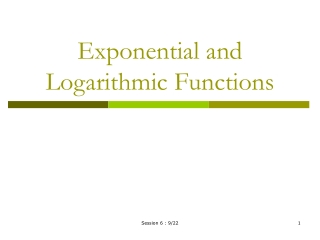DownloadDownload PresentationExponential and Logarithmic Functions

# Exponential and Logarithmic Functions

Télécharger la présentation## Exponential and Logarithmic Functions

- - - - - - - - - - - - - - - - - - - - - - - - - - - E N D - - - - - - - - - - - - - - - - - - - - - - - - - - -
##### Presentation Transcript

1. Exponential and Logarithmic Functions Session 6 : 9/22

2. Exponential Functions • Definition: If a is some number greater than 0, and a = 1, then the exponential function with base a is: Examples: Session 6 : 9/22

3. Properties of Exponents • If a and b are positive numbers: Session 6 : 9/22

4. Graphs of Exponential Functions • Point plotting or graphing tool • If base is raised to positive x, function is an increasing exponential. If base is raised to negative x, function is a decreasing exponential • If a>1 and to a (+)x : Increasing Exponential • If a<1 and to a (+)x : Decreasing Exponential • If a>1 and to a (-)x: Decreasing Exponential • If a<1 and to a (-)x: Increasing Exponential Session 6 : 9/22

5. Session 6 : 9/22

6. Sketching an Exponential • Find horizontal asymptote and plot several points • How do we find horizontal asymptote? • Take the limit as x approaches infinite (for decreasing exponentials) or negative infinite (for increasing exponentials) Session 6 : 9/22

7. INCREASING EXPONENTIAL 8 x - Asymptote for increasing exponential function 8 x + Asymptote for decreasing exponential function Session 6 : 9/22

8. Natural Exponential Functions • In calculus, the most convenient (or natural) base for an exponential function is the irrational number e (will become more obvious once we start trying to differentiate/integrate…) • e ≈ 2.718 • Simplest Natural Exponential: Session 6 : 9/22

9. Graph of the Natural Exponential Function Session 6 : 9/22

10. Exponential Growth • Exponential functions (particularly natural exponentials) are commonly used to model growth of a quantity or a population • What growth is unrestricted, can be described by a form of the standard exponential function (probably will have multiplying constants, slight changes…): • When growth is restricted, growth may be best described by the logistic growth function: Where a, b, and k are constants defined for a given population under specified conditions. Session 6 : 9/22

11. Comparing Exponential v. Logistic Growth Function y Exponential Logistic Growth Function x Session 6 : 9/22

12. Derivatives of The Natural Exponential Function • From now on, ‘Exponential Function’ will imply an function with base e • Previously, we said that e is the most convenient base to use in calculus. Why? • Very simple derivative! Chain rule, where u is a function of x Session 6 : 9/22

13. What does this mean graphically? For the function the slope at any point x is given by the derivative slope = e2 slope =e1 slope =e0=1 1 2 Session 6 : 9/22

14. Examples: Session 6 : 9/22

15. Logarithmic Functions • Review of ‘log’ If no base specified, log10 Session 6 : 9/22

16. The Natural Log • Natural Log=loge=ln • Definition of the natural log: The natural logarithmic function, denoted by ln(x), is defined as: Why? Session 6 : 9/22

17. Important Properties of Logarithmic Functions Natural log is inverse of exponential Exponential is inverse of natural log Session 6 : 9/22

18. Examples: • Solve the following logarithmic functions for x • Simplify the following: Session 6 : 9/22

19. Examples: Solving Exponential and Logarithmic Equations Session 6 : 9/22

20. Example: Doubling Time • For an account with initial balance P, the function for the account balance (A) after t years (with annual interest rate r compounded continuously) is given by: Find an expression for the time at which the account balance has doubled. Session 6 : 9/22

21. Derivative of logarithmic functions: Where u is a function of x Session 6 : 9/22

22. Examples Session 6 : 9/22

23. Exponential Growth and Decay • Law of exponential growth and decay: If y is a positive quantity whose rate of change with respect to time is proportional to the quantity present at any time t, then y is described by: Where C is the initial value k is the constant of proportionality (often rate constant) If k > 0: Exponential Growth If k < 0: Exponential Decay Session 6 : 9/22

24. Example: • Modeling population growth: • A researcher is trying to develop an equation to describe bacterial growth, and knows that it will follow the fundamental equation for exponential growth. The following data is available: • At t=2 hours, there are 1x106 cells • At t=8 hours, there are 5x108 cells • Write an equation for the exponential growth of bacterial cells by the following steps: • 1. Find k • 2. Find C using the solution for k • 3. Write the full model by plugging in C and k values. • Find the time at which the population is double that of the initial population. Session 6 : 9/22